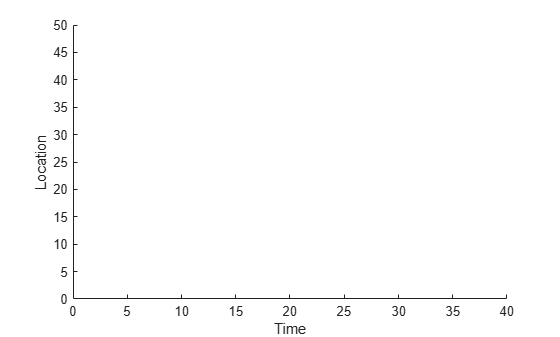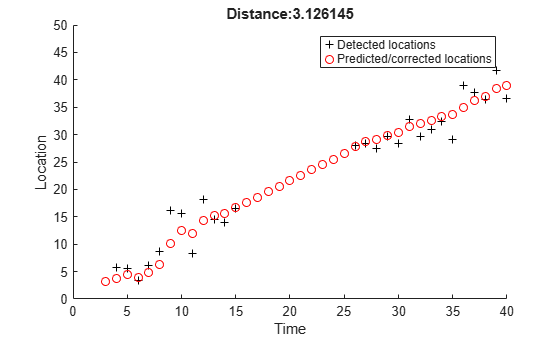# distance

Confidence value of measurement

## Syntax

``d = distance(kalmanFilter,zmatrix)``

## Description

example

````d = distance(kalmanFilter,zmatrix)` computes a distance between the location of a detected object and the predicted location by the Kalman filter object. This distance computation takes into account the covariance of the predicted state and the process noise. The `distance` function can only be called after the `predict` function.Use the `distance` function to find the best matches. The computed distance values describe how a set of measurements matches the Kalman filter. You can thus select a measurement that best fits the filter. This strategy can be used for matching object detections against object tracks in a multiobject tracking problem. This distance computation takes into account the covariance of the predicted state and the process noise.```

## Examples

collapse all

Track the location of a physical object moving in one direction.

Generate synthetic data which mimics the 1-D location of a physical object moving at a constant speed.

`detectedLocations = num2cell(2*randn(1,40) + (1:40));`

Simulate missing detections by setting some elements to empty.

```detectedLocations{1} = []; for idx = 16: 25 detectedLocations{idx} = []; end```

Create a figure to show the location of detections and the results of using the Kalman filter for tracking.

```figure; hold on; ylabel('Location'); ylim([0,50]); xlabel('Time'); xlim([0,length(detectedLocations)]);```Create a 1-D, constant speed Kalman filter when the physical object is first detected. Predict the location of the object based on previous states. If the object is detected at the current time step, use its location to correct the states.

```kalman = []; for idx = 1: length(detectedLocations) location = detectedLocations{idx}; if isempty(kalman) if ~isempty(location) stateModel = [1 1;0 1]; measurementModel = [1 0]; kalman = vision.KalmanFilter(stateModel,measurementModel,'ProcessNoise',1e-4,'MeasurementNoise',4); kalman.State = [location, 0]; end else trackedLocation = predict(kalman); if ~isempty(location) plot(idx, location,'k+'); d = distance(kalman,location); title(sprintf('Distance:%f', d)); trackedLocation = correct(kalman,location); else title('Missing detection'); end pause(0.2); plot(idx,trackedLocation,'ro'); end end legend('Detected locations','Predicted/corrected locations');```Use Kalman filter to remove noise from a random signal corrupted by a zero-mean Gaussian noise.

Synthesize a random signal that has value of 1 and is corrupted by a zero-mean Gaussian noise with standard deviation of 0.1.

```x = 1; len = 100; z = x + 0.1 * randn(1,len);```

Remove noise from the signal by using a Kalman filter. The state is expected to be constant, and the measurement is the same as state.

```stateTransitionModel = 1; measurementModel = 1; obj = vision.KalmanFilter(stateTransitionModel,measurementModel,'StateCovariance',1,'ProcessNoise',1e-5,'MeasurementNoise',1e-2); z_corr = zeros(1,len); for idx = 1: len predict(obj); z_corr(idx) = correct(obj,z(idx)); end```

Plot results.

```figure, plot(x * ones(1,len),'g-'); hold on; plot(1:len,z,'b+',1:len,z_corr,'r-'); legend('Original signal','Noisy signal','Filtered signal');```## Input Arguments

collapse all

Kalman filter object.

Location of a detected object, specified as an N-column matrix. Each row matrix contains a measurement vector. The `distance` function returns a row vector where each distance element corresponds to the measurement input.

collapse all

### Distance Equation

`$d\left(z\right)={\left(z-Hx\right)}^{T}{\sum }_{}^{-1}\left(z-Hx\right)+\mathrm{ln}|\sum |$`

Where $\Sigma =HP{H}^{T}+R$ and $|\Sigma |$ is the determinant of $\Sigma$. You can then find the best matches by examining the returned distance values.